CHAPTER 1. Mathematics Division of Positive and Negative Numbers The quotient of two positive numbers is always positive. The quotient of two negative numbers is always positive. The quotient of a positive and negative number is always negative.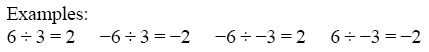Powers The power (or exponent) of a number is a shorthand method of indicating how many times a number, called the base, is multiplied by itself. For example, 34 means “3 to the power of 4." That is, 3 multiplied by itself 4 times. The 3 is the base and 4 is the power.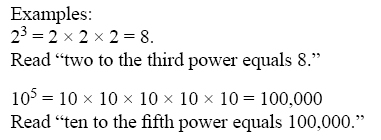Special Powers Squared. When a number has a power of 2, it is commonly referred to as “squared." For example, 72 is read as “seven squared" or “seven to the second power." To remember this, think about how a square has two dimensions: length and width. Cubed. When a number has a power of 3, it is commonly referred to as “cubed." For example, 73 is read as “seven cubed" or “seven to the third power." To remember this, think about how a cube has three dimensions: length, width, and depth. Power of Zero. Any non-zero number raised to the zero power always equals 1. Example:             70 = 1 1810 = 1 (-24)0 = 1 Negative Powers A number with a negative power equals its reciprocal with the same power made positive. Example: The number 2-3 is read as “2 to the negative 3rd power," and is calculated by: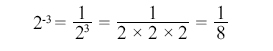When using a calculator to raise a negative number to a power, always place parentheses around the negative number (before raising it to a power) so that the entire number gets raised to the power. Law of Exponents When multiplying numbers with powers, the powers can be added as long as the bases are the same.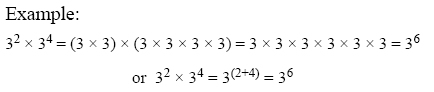When dividing numbers with powers, the powers can be subtracted as long as the bases are the same.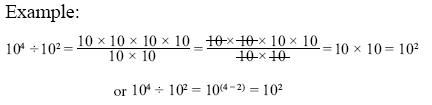Powers of Ten Because we use the decimal system of numbers, powers of ten are frequently seen in everyday applications. For example, scientific notation uses powers of ten. Also, many aircraft drawings are scaled to powers of ten. Figure 1-9 gives more information on the powers of ten and their values.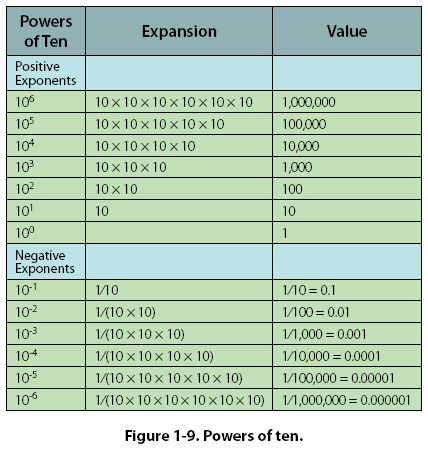Roots A root is a number that when multiplied by itself a specified number of times will produce a given number. The two most common roots are the square root and the cube root. For more examples of roots, see the chart in Figure 1-10, Functions of Numbers (on page 1-14).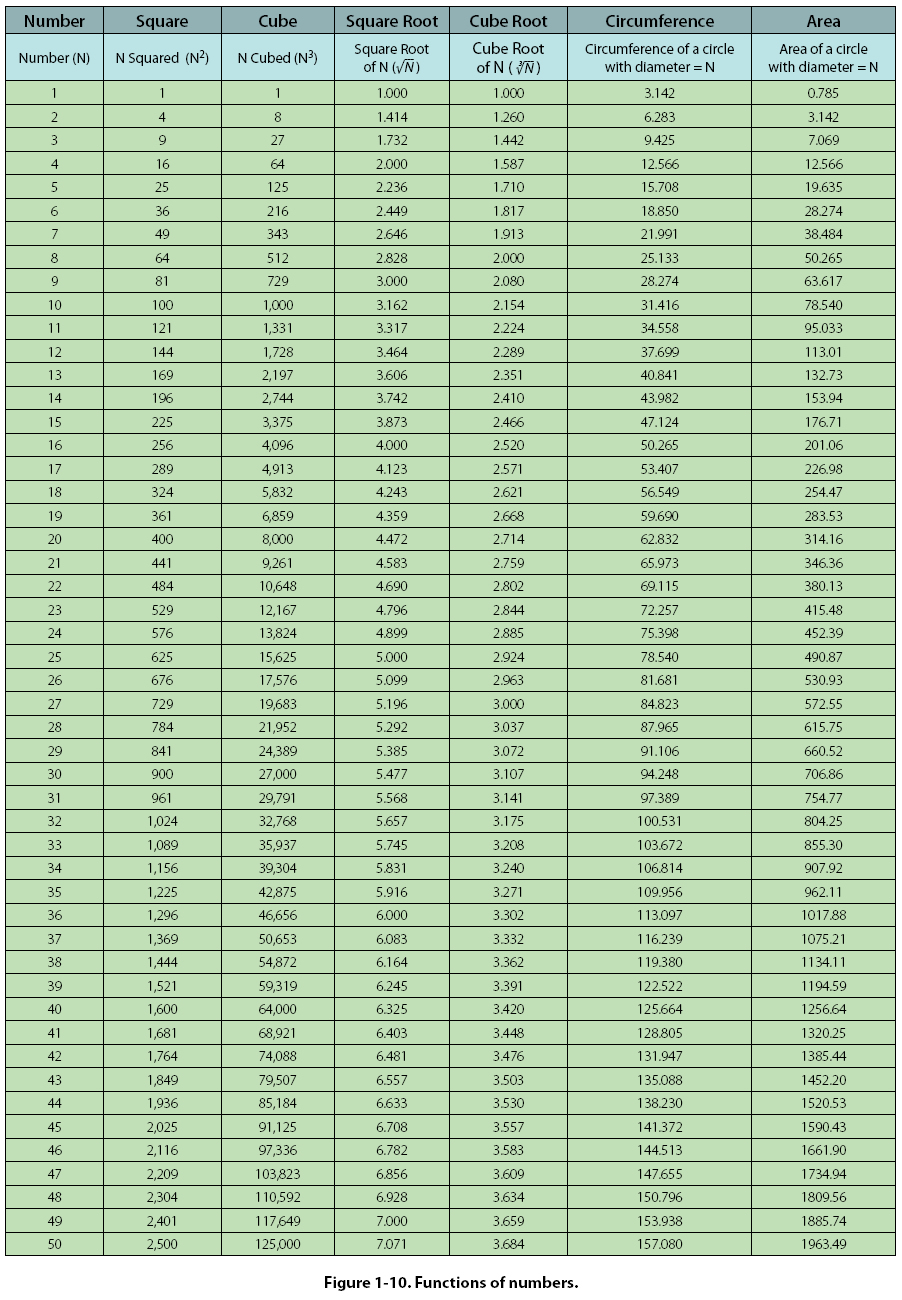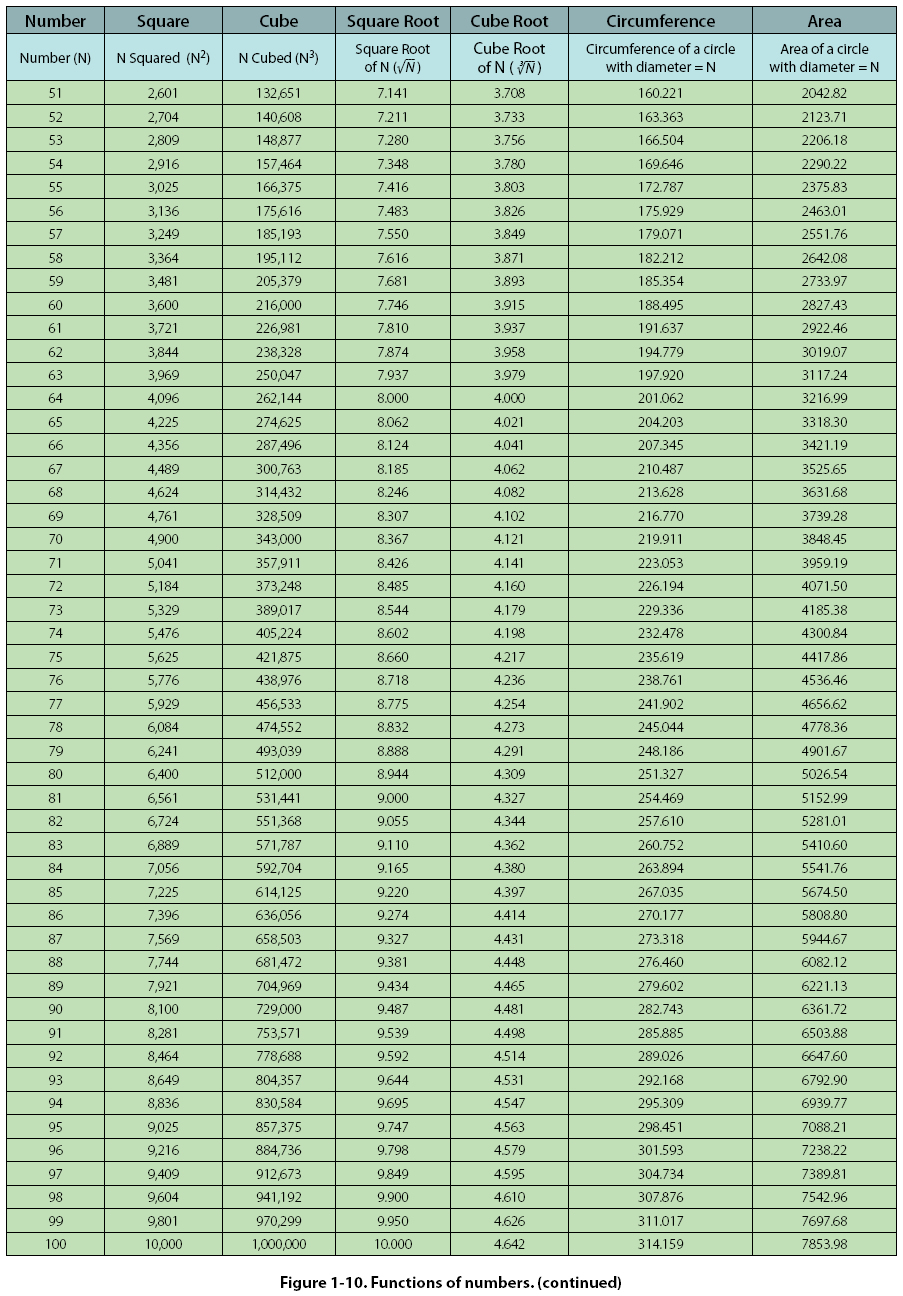©AvStop Online Magazine                                                                                                                                                      Contact Us              Return To Books